# Data Visualization in R using ggplot2

For the purpose of data visualization, R offers various methods through inbuilt graphics and powerful packages such as ggolot2. Former helps in creating simple graphs while latter assists in creating customized professional graphs. In this article we will try to learn how various graphs can be made and altered using ggplot2 package.Data Visualization with R

What is ggplot2?

ggplot2 is a robust and a versatile R package, developed by the most well known R developer, Hadley Wickham, for generating aesthetic plots and charts.

The ggplot2 implies "Grammar of Graphics" which believes in the principle that a plot can be split into the following basic parts -
Plot = data + Aesthetics + Geometry
1. data refers to a data frame (dataset).
2. Aesthetics indicates x and y variables. It is also used to tell R how data are displayed in a plot, e.g. color, size and shape of points etc.
3. Geometry refers to the type of graphics (bar chart, histogram, box plot, line plot, density plot, dot plot etc.)
Apart from the above three parts, there are other important parts of plot -
1. Faceting implies the same type of graph can be applied to each subset of the data. For example, for variable gender, creating 2 graphs for male and female.
2. Annotation lets you to add text to the plot.
3. Summary Statistics allows you to add descriptive statistics on a plot.
4. Scales are used to control x and y axis limits

Why ggplot2 is better?
• Excellent themes can be created with a single command.
• Its colors are nicer and more pretty than the usual graphics.
• Easy to visualize data with multiple variables.
• Provides a platform to create simple graphs providing plethora of information.

The table below shows common charts along with various important functions used in these charts.
Important Plots Important Functions
Scatter Plot geom_point(), geom_smooth(), stat_smooth()
Bar Chart geom_bar(), geom_errorbar()
Histogram geom_histogram(), stat_bin(), position_identity(), position_stack(), position_dodge()
Box Plot geom_boxplot(), stat_boxplot(), stat_summary()
Line Plot geom_line(), geom_step(), geom_path(), geom_errorbar()
Pie Chart coord_polar()

Datasets

In this article, we will use three datasets - 'iris' , 'mpg' and 'mtcars' datasets available in R.

1. The 'iris' data comprises of 150 observations with 5 variables. We have 3 species of flowers: Setosa, Versicolor and Virginica and for each of them the sepal length and width and petal length and width are provided.

2. The 'mtcars' data consists of fuel consumption (mpg) and 10 aspects of automobile design and performance for 32 automobiles. In order words, we have 32 observations and 11 different variables:
1. mpg Miles/(US) gallon
2. cyl Number of cylinders
3. disp Displacement (cu.in.)
4. hp Gross horsepower
5. drat Rear axle ratio
6. wt Weight (1000 lbs)
7. qsec 1/4 mile time
8. vs V/S
9. am Transmission (0 = automatic, 1 = manual)
10. gear Number of forward gears
11. carb Number of carburetors

3. The 'mpg' data consists of 234 observations and 11 variables.

First we need to install package in R by using command install.packages( ).
#installing package
install.packages("ggplot2")
library(ggplot2)
Once installation is completed, we need to load the package so that we can use the functions available in the ggplot2 package. To load the package, use command library( )

Histogram, Density plots and Box plots are used for visualizing a continuous variable.

## Creating Histogram:

Firstly we consider the iris data to create histogram and scatter plot.
# Considering the iris data.
# Creating a histogram
ggplot(data  = iris, aes( x = Sepal.Length)) + geom_histogram( )
Here we call ggplot( ) function, the first argument being the dataset to be used.

1. aes( ) i.e. aesthetics we define which variable will be represented on the x- axis; here we consider 'Sepal.Length'
2. geom_histogram( ) denotes we want to plot a histogram.Histogram in R

To change the width of bin in the histograms we can use binwidth in geom_histogram( )
ggplot(data  = iris, aes(x = Sepal.Length)) + geom_histogram(binwidth=1)

One can also define the number of bins being wanted, the binwidth in that case will be adjusted automatically.
ggplot(data = iris , aes(x=Sepal.Length)) + geom_histogram(color="black", fill="white", bins = 10

Using  color = "black" and fill = "white" we are denoting the boundary colors and the inside color of the bins respectively.

### How to visualize various groups in histogram

ggplot(iris, aes(x=Sepal.Length, color=Species)) + geom_histogram(fill="white", binwidth = 1)Histogram depicting various species

#### Creating Density Plot

Density plot is also used to present the distribution of a continuous variable.
ggplot(iris, aes( x = Sepal.Length)) + geom_density( )
geom_density( ) function is for displaying density plot.Density Plot

#### How to show various groups in density plot

ggplot(iris, aes(x=Sepal.Length, color=Species)) + geom_density( )Density Plot by group

Creating Bar and Column Charts :
Bar and column charts are probably the most common chart type. It is best used to compare different values.

Now mpg data will be used for creating the following graphics.
ggplot(mpg, aes(x= class)) + geom_bar()
Here we are trying to create a bar plot for number of cars in each class using geom_bar( ).Column Chart using ggplot2

Using coord_flip( ) one can inter-change x and y axis.
ggplot(mpg, aes(x= class)) + geom_bar() + coord_flip()Bar Chart

How to add or modify Main Title and Axis Labels

The following functions can be used to add or alter main title and axis labels.
1. ggtitle("Main title"): Adds a main title above the plot
2. xlab("X axis label"): Changes the X axis label
3. ylab("Y axis label"): Changes the Y axis label
4. labs(title = "Main title", x = "X axis label", y = "Y axis label"): Changes main title and axis labels
p = ggplot(mpg, aes(x= class)) + geom_bar()
p + labs(title = "Number of Cars in each type", x = "Type of car", y = "Number of cars")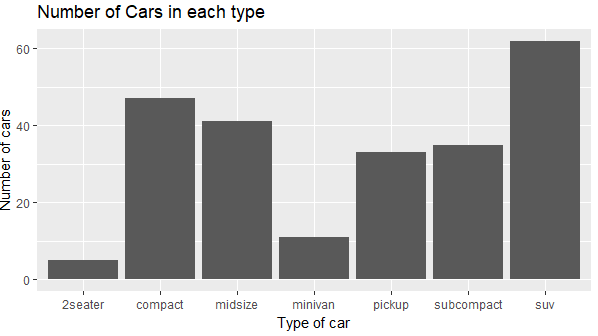Title and Axis Labels
p = ggplot(mpg, aes(x= class)) + geom_bar()
p = p + labs(title = "Number of Cars in each type", x = "Type of car", y = "Number of cars")
p + geom_text(stat='count', aes(label=..count..), vjust=-0.25)
geom_text() is used to add text directly to the plot. vjust is to adjust the position of data labels in bar.Add Data Labels in Bar

How to reorder Bars
Using stat="identity" we can use our derived values instead of count.
library(plyr)
library(dplyr)
count(mpg,class) %>% arrange(-n) %>%
mutate(class = factor(class,levels= class)) %>%
ggplot(aes(x=class, y=n)) + geom_bar(stat="identity")
The above command will firstly create a frequency distribution for the type of car and then arrange it in descending order using arrange(-n). Then using mutate( )  we modify the 'class' column to a factor with levels 'class' and hence plot the bar plot using geom_bar( ).Change order of bars

Here, bar of SUV appears first as it has maximum number of cars. Now bars are ordered based on frequency count.

Showing Mean of Continuous Variable by Categorical Variable
df = mpg %>% group_by(class) %>% summarise(mean = mean(displ)) %>%
arrange(-mean) %>% mutate(class = factor(class,levels= class))

p = ggplot(df, aes(x=class, y=mean)) + geom_bar(stat="identity")
p + geom_text(aes(label = sprintf("%0.2f", round(mean, digits = 2))),
vjust=1.6, color="white", fontface = "bold", size=4)

Now using dplyr library we create a new dataframe 'df' and try to plot it.
Using group_by we group the data according to various types of cars and summarise enables us to find the statistics (here mean for 'displ' variable) for each group. To add data labels (with 2 decimal places) we use geom_text( )Customized BarPlot
Creating Stacked Bar Chart
p <- ggplot(data=mpg, aes(x=class, y=displ, fill=drv))
p + geom_bar(stat = "identity")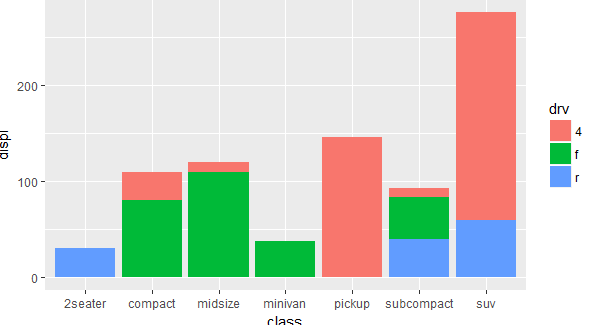Stacked BarPlot
p + geom_bar(stat="identity", position=position_dodge())Stacked - Position_dodge

Creating BoxPlot

Using geom_boxplot( ) one can create a boxplot.

To create different boxplots for 'disp' for different levels of x we can define aes(x = cyl, y = disp)
mtcars\$cyl = factor(mtcars\$cyl)
ggplot(mtcars, aes(x=cyl, y=disp)) + geom_boxplot()
We can see one outlier for 6 cylinders.

To create a notched boxplot we write notch = TRUE
ggplot(mtcars, aes(x=cyl, y=disp)) + geom_boxplot(notch = TRUE)Notched Boxplot

Scatter Plot

A scatterplot is used to graphically represent the relationship between two continuous variables.
# Creating a scatter plot denoting various species.
ggplot(data = iris, aes( x = Sepal.Length, y = Sepal.Width,shape = Species, color = Species)) + geom_point()
We plot the points using geom_point( ). In the aesthetics we define that x axis denotes sepal length, y axis denotes sepal width; shape = Species and color = Species denotes that different shapes and different sizes should be used for each particular specie of flower.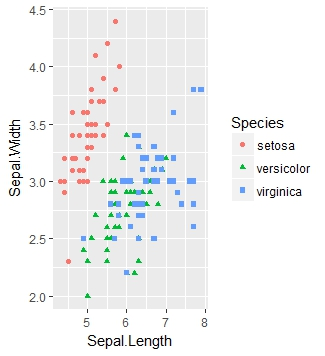Scatter Plot
Scatter plots are constructed using geom_point( )
# Creating scatter plot for automatic cars denoting different cylinders.
ggplot(data = subset(mtcars,am == 0),aes(x = mpg,y = disp,colour = factor(cyl))) + geom_point()Scatter plot denotingvarious levels of cyl
We use subset( ) function to select only those cars which have am = 0; paraphrasing it; we are considering only those cars which are automatic. We plot the displacement corresponding to mileage and for different cylinders we are using various colors. Also factor(cyl) transforms our continuous variable cylinder to a factor.
# Seeing the patterns with the help of geom_smooth.
ggplot(data = mtcars, aes(x = mpg,y = disp,colour = hp))  + geom_point() + geom_smooth()
In the above command we try to plot mileage (mpg) and displacement (disp) and variation in colors denote the varying horsepower(hp) .  geom_smooth( ) is used to determine what kind of pattern is exhibited by the points.
In a similar way we can use geom_line( ) to plot another line on the graph:
# Plotting the horsepower using geom_line
ggplot(data = mtcars, aes(x = mpg,y = disp,colour = hp))  + geom_point(size = 2.5) + geom_line(aes(y = hp))
Here in geom_point we have added an optional argument size = 2.5 denoting the size of the points. geom_line( ) creates a line. Note that we have not provided any aesthetics for x axis in geom_line, it means that it will plot the horsepower(hp) corresponding to mileage(mpg) only.

Modifying the axis labels and appending the title and subtitle
#Adding title or changing the labels
ggplot(mtcars,aes(x = mpg,y = disp)) + geom_point() + labs(title = "Scatter plot")
#Alternatively
ggplot(mtcars,aes(x = mpg,y = disp)) + geom_point() + ggtitle(label = "Scatter plot")
ggplot(mtcars,aes(x = mpg,y = disp)) + geom_point() + ggtitle(label = "Scatter plot",
subtitle = "mtcars data in R")Adding title and subtitle to plots
Here using labs( ) we can change the title of our legend or ggtitle we can assign our graph some title. If we want to add some title or sub-title to our graph thus we can use ggtitle( ) where the first argument is our 'main title' and second argument is our subtitle.
a <- ggplot(mtcars,aes(x = mpg, y = disp, color = factor(cyl))) + geom_point()
a
#Changing the axis labels.
a + labs(color = "Cylinders")
a + labs(color = "Cylinders") + xlab("Mileage") + ylab("Displacement")
We firstly save our plot to 'a' and thus we make the alterations.
Note that in the labs command we are using color = "Cylinders" which changes the title of our legend.
Using the xlab and ylab commands we can change the x and y axis labels respectively. Here our x axis label is 'mileage' and y axis label is 'displacement'
#Combining it all
a + labs(color = "Cylinders") + xlab("Mileage") + ylab("Displacement") + ggtitle(label = "Scatter plot", subtitle = "mtcars data in R")
In the above plot we can see that the labels on x axis,y axis and legend have changed; the title and subtitle have been added and the points are colored, distinguishing the number of cylinders.

Playing with themes
Themes can be used in ggplot2 to change the backgrounds,text colors, legend colors and axis texts.
Firstly we save our plot to 'b' and hence create the visualizations by manipulating 'b'. Note that in aesthetics we have written mpg, disp which automatically plots mpg on x axis and disp on y axis.
#Changing the themes.
b <- ggplot(mtcars,aes(mpg,disp)) + geom_point()  + labs(title = "Scatter Plot")
#Changing the size and color of the Title and the background color.
b + theme(plot.title = element_text(color = "blue",size = 17),plot.background = element_rect("orange"))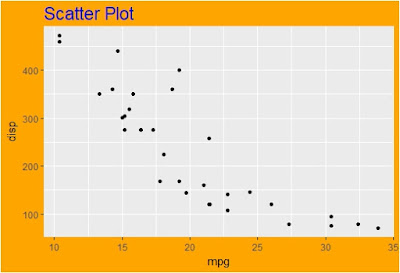Plot background color changed.
We use theme( ) to modify the the plot title and background. plot.title is an element_text( ) object in which we have specified the color and size of our title. Utilizing plot.background which is an element_rect( ) object we can specify the color of our background.
ggplot2( ) offers by default themes with background panel design colors being changed automatically. Some of them are theme_gray, theme_minimal, theme_dark etc.
b + theme_minimal( )
We can observe horizontal and vertical lines behind the points. What if we don't need them? This can be achieved via:
#Removing the lines from the background.
b + theme(panel.background = element_blank())

Setting panel.background = element_blank( ) with no other parameter can remove those lines and color from the panel.
#Removing the text from x and y axis.
b + theme(axis.text = element_blank())
b + theme(axis.text.x = element_blank())
b + theme(axis.text.y = element_blank())
To remove the text from both the axis we can use axis.text = element_blank( ). If we want to remove the text only from particular axis then we need to specify it.
Now we save our plot to c and then make the changes.
#Changing the legend position
c <- ggplot(mtcars,aes(x = mpg, y = disp, color = hp)) +labs(title = "Scatter Plot") + geom_point()
c +  theme(legend.position = "top")
If we want to move the legend then we can specify legend.position as "top" or "bottom" or "left" or "right".
Finally combining all what we have learnt in themes we create the above plot where the legend is placed at bottom, plot title is in forest green color, the background is in yellow and no text is displayed on both the axis.
#Combining everything.
c + theme(legend.position = "bottom", axis.text = element_blank()) +
theme(plot.title = element_text(color = "Forest Green",size = 17),plot.background = element_rect("Yellow"))Scatter Plot

Changing the color scales in the legend
In ggplot2, by default the color scale is from dark blue to light blue. It might happen that we wish to innovate the scales by changing the colors or adding new colors. This can be done successfuly via scale_color_gradient function.
c + scale_color_gradient(low = "yellow",high = "red")
Suppose we want the colors to vary from yellow to red; yellow denoting the least value and red denoting the highest value; we set low = "yellow" and high = "red". Note that in the legend it takes the scale to be started from 0 and not the minimum value of the series.
What if we want 3 colors?
c + scale_color_gradient2(low = "red",mid = "green",high = "blue")
To serve the purpose of having 3 colors in the legend we use scale_color_gradient2 with low = "red",mid = "green" and high = "blue" means it divides the entire range(Starting from 0) to the maximum observation in 3 equal parts, with first part being shaded as red, central part as green and highest part as blue.
c + theme(legend.position = "bottom") + scale_color_gradientn(colours = c("red","forest green","white","blue"))
If we want more than 3 colors to be represented by our legend we can utilize scale_color_gradientn( ) function and the argument colors will be a vector starting where 1st element denotes the color of the 1st part, 2nd color denotes the color of 2nd part etc.

Changing the breaks in the legend.
It can be seen that the legend for continuous variable starts from 0.
Suppose we want the breaks to be: 50,125,200,275 and 350, we use seq(50,350,75) where 50 denotes the least number, 350 is the maximum number in the sequence and 75 is the difference between 2 consecutive numbers.
#Changing the breaks in the legend
c + scale_color_continuous(name = "horsepower", breaks = seq(50,350,75), labels = paste(seq(50,350,75),"hp"))
In scale_color_continuous we set the breaks as our desired sequence, and can change the labels if we want. Using paste function our sequence is followed by the word "hp" and name = "horsepower" changes the name of our legend.

Changing the break points and color scale of the legend together.
Let us try changing the break points and the colors in the legend together by trial and error.
#Trial 1 : This one is wrong
c + scale_color_continuous( breaks = seq(50,350,75)) +
We can refer to trial1 image for the above code which can be found below. Notice that the color scale is blue to red as desired but the breaks have not changed.
#Trial 2: Next one is wrong.
c  +  scale_color_gradient(low = "blue",high = "red") +
scale_color_continuous( breaks = seq(50,350,75))
trial2 image is the output for the above code. Here the color scale has not changed but the breaks have been created.trial1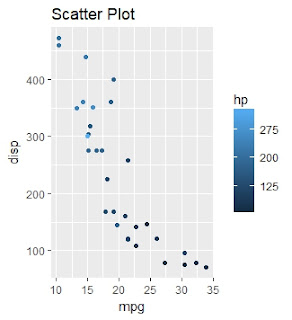trial2

What is happening? The reason for this is that we cannot have 2 scale_color functions for a single graph. If there are multiple scale_color_ functions then R overwrites the other scale_color_ functions by the last scale_color_ command it has received.
In trial 1, scale_color_gradient overwrites the previous scale_color_continuous command. Similarly in trial 2, scale_color_continuous overwrites the previous scale_color_gradient command.

The correct way to do is to define the arguments in one function only.
c + scale_color_continuous(name = "horsepower", breaks = seq(50,350,75), low = "red", high = "black") + theme(panel.background = element_rect("green"),
plot.background = element_rect("orange"))
Here low = "red" and high = "black" are defined in scale_color_continuous function along with the breaks.

Changing the axis cut points

We save our initial plot to 'd'.
d <- ggplot(mtcars,aes(x = mpg,y = disp)) + geom_point(aes(color = factor(am)))  +
xlab("Mileage") + ylab("Displacement") +
theme(panel.background = element_rect("black") , plot.background = element_rect("pink"))
To change the axis cut points we use scale_(axisname)_continuous.
d +  scale_x_continuous(limits = c(2,4)) + scale_y_continuous(limits = c(15,30))
To change the x axis limits to 2 to 4, we use scale_x_continuous and my 'limits' is a vector defining the upper and lower limits of the axis. Likewise, scale_y_continuous set the least cut off point to 15 and highest cut off point of y axis to 30.

d + scale_x_continuous(limits = c(2,4),breaks = seq(2,4,0.25)) +
scale_y_continuous(limits = c(15,30),breaks = seq(15,30,3))
We can also add another parameter 'breaks' which will need a vector to specify all the cut of points of the axis. Here we create a sequence of 2,2.5,3,3.5,4 for x axis and for y axis the sequence is 15,18,21,...,30.

Faceting.
Faceting is a technique which is used to plot the graphs for the data corresponding to various categories of a particular variable. Let us try to understand it via an illustration:

facet_wrap function is used for faceting where the after the tilde(~) sign we define the variables on which we want the classification.Faceting for carb
We see that there are 6 categories of "carb". Faceting creates 6 plots between mpg and disp; where the points correspond to the categories.
We can mention the number of rows we need for faceting.
# Control the number of rows and columns with nrow and ncol
ggplot(mtcars, aes(mpg, disp)) +  geom_point() +  facet_wrap(~carb,nrow = 3)
Here an additional parameter nrow =  3 depicts that in total all the graphs should be adjusted in 3 rows.

Faceting using multiple variables.
Faceting can be done for various combinations of carb and am.
# You can facet by multiple variables
ggplot(mtcars, aes(mpg, disp)) + geom_point() + facet_wrap(~carb + am)
#Alternatively
ggplot(mtcars, aes(mpg, disp)) + geom_point() + facet_wrap(c("carb","am"))
There are 6 unique 'carb' values and 2 unique 'am' values thus there could be 12 possible combinations but we can get only 9 graphs, this is because for remaining 3 combinations there is no observation.
It might be puzzling to grasp which the level of am and carb specially when the labels ain't provided. Accordingly we can label the variables.
# Use the `labeller` option to control how labels are printed:
ggplot(mtcars, aes(mpg, disp)) +  geom_point() +  facet_wrap(~carb  + am, labeller = "label_both")facet_wrap in multiple variables.
R provides facet_grid( ) function which can be used to faced in two dimensions.
z <- ggplot(mtcars, aes(mpg, disp)) + geom_point()
We store our basic plot in 'z' and thus we can make the additions:
z + facet_grid(. ~ cyl)   #col
z + facet_grid(cyl ~ .)   #row
z + facet_grid(gear ~ cyl,labeller = "label_both")  #row and colusing facet_grid( )
In facet_grid(.~cyl), it facets the data by 'cyl' and the cylinders are represented in columns. If we want to represent 'cyl' in rows, we write facet_grid(cyl~.). If we want to facet according to 2 variables we write facet_grid(gear~cyl) where gears are represented in rows and 'cyl' are illustrated in columns.

Using ggplot2 we can define what are the different values / labels for all the points. This can be accomplished by using geom_text( )
ggplot(mtcars, aes(x= mpg,y = disp)) + geom_point() +
geom_text(aes(label = am))
In geom_text we provide aes(label = am) which depicts that for all the points the corresponding levels of "am" should be shown.
In the graph it can be perceived that the labels of 'am' are overlapping with the points. In some situations it may become difficult to read the labels when there are many points. In order to avoid this we use geom_text_repel function in 'ggrepel' library.
require(ggrepel)
ggplot(mtcars, aes(x= mpg,y = disp)) + geom_point() +
geom_text_repel(aes(label = am))
We load the library ggrepel using require( ) function. If we don't want the text to overlap we use geom_text_repel( ) instead of geom_text( ) of ggplot2 , keeping the argument aes(label = am).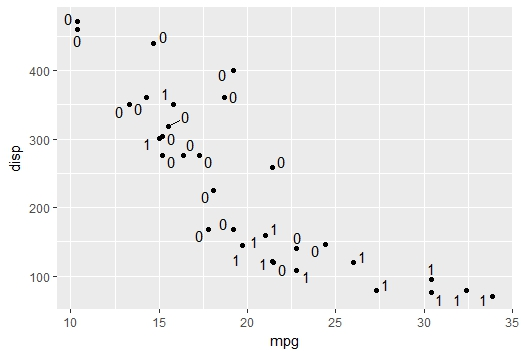geom_text_repel
Special thanks to  Ekta Aggarwal for her contribution in this article. She is a co-author of this article. She is a Data Science enthusiast, currently in the final year of her post graduation in statistics from Delhi University.Share
Related PostsDeepanshu founded ListenData with a simple objective - Make analytics easy to understand and follow. He has over 10 years of experience in data science. During his tenure, he has worked with global clients in various domains like Banking, Insurance, Private Equity, Telecom and Human Resource.

6 Responses to "Data Visualization in R using ggplot2"
1.Really informative. Clean code and wonderful plot. I like the table at beginning.

2.thanks a lot !!

3.It's really useful.Thanks alot!!!

4.how to create a boxplot using one categorical variable and two numeric variable in r

1.Animals <- c("giraffes", "orangutans", "monkeys")

SF_Zoo <- c(20, 14, 23,23,11,12)

LA_Zoo <- c(12, 18, 29,12,18,29)

dataPlotLy <- data.frame(Animals, SF_Zoo, LA_Zoo)

Fin <-aggregate(. ~ Animals, dataPlotLy , sum)

Regarding the above how to create a boxplot using one categorical variable and two numeric variable in r

5.In the section "How to reorder bars", the code given produces the following error for me:
Error in UseMethod("as.quoted") :
no applicable method for 'as.quoted' applied to an object of class "function"